### Home > CALC > Chapter 8 > Lesson 8.2.3 > Problem8-87

8-87.
1. Let g(x) =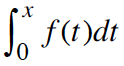where f is shown at right. Homework Help ✎

1. Find g(2) and g(4).

2. Is g(−2) =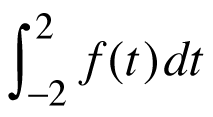?

3. Expressin terms of g.

4. Is g differentiable over the interval −2 < x < 6 ? Explain.

5. Find all values of x on the interval −2 < x < 6 where g has a relative maximum.

6. Find the line tangent to g at x = 4.

7. Find all values of x on the interval −2 < x < 6 where g has a point of inflection.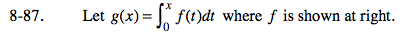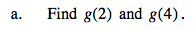$\text{Since }g(x)\text{ was defined as }\int_{0}^{x}f(t)dt,$

$g(2)=\int_{0}^{2}f(t)dt$

$g(4)=\int_{0}^{4}f(t)dt$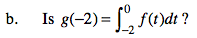Consider the symmetry of the f(t) between t = −2 and t = 2.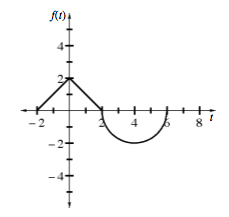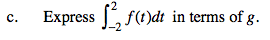$\int_{-2}^{2}f(t)dt=kg(a)$

What is k? What is a?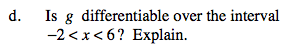Points of NON-differentiablity include cusps, endpoints, jumps, holes and vertical tangents.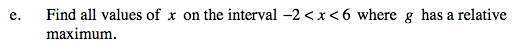Recall that a local maximum exists where the derivative changes from positive to negative. This could happen where f '(x) = 0 or where f '(x) = DNE.

Notice that g'(x) = f(x); after all, the derivative of an integral is the original function.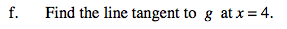What is the slope of g(x) at x = 4 (see second hint in part (e))? What is the y-value?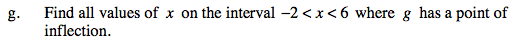Concavity is the slope of the slope. So inflection points are where the slope of the slope changes.